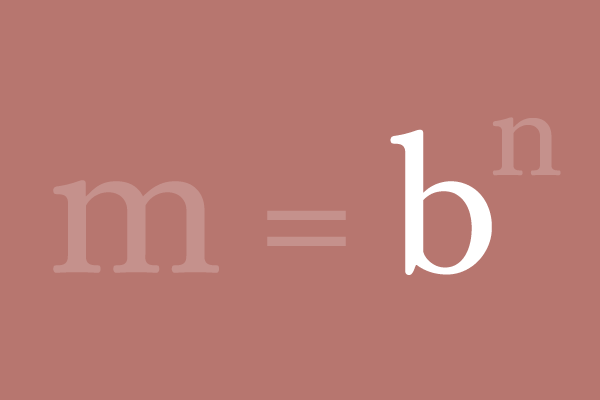# Base quantity

A quantity that splits another quantity as its factors is called the base.## Introduction

A quantity can be expressed in product form on the basis of another quantity and the product form expression can be written simply in exponential notation as per exponentiation. The quantity that is considered to split a quantity as a product of its terms is called the base of exponential form.

### Example

$81$ is a number and express it in product form on the basis of another number $3$ by factorization.

$81 \,=\, 3 \times 3 \times 3 \times 3$

According to exponentiation, write the product form expression in exponential notation.

$81 \,=\, 3^4$

On the basis of number $3$, the number $81$ is expressed as factors of $3$. Hence, the number $3$ is called the base of the exponential notation for the number $81$.

#### More Examples

Observe the following examples to understand what exactly the base is in exponential notation.

$(1)\,\,\,\,\,\,\,$ $8 = 2 \times 2 \times 2 = 2^3$
$\,\,\,\,\,\,\,\,\,\,\,\,\,\,\,$ In this example, $2$ is the base of the exponential term.

$(2)\,\,\,\,\,\,\,$ $16 = 4 \times 4 = 4^2$
$\,\,\,\,\,\,\,\,\,\,\,\,\,\,\,$ In this example, $4$ is the base of the exponential term.

$(3)\,\,\,\,\,\,\,$ $3125 = 5 \times 5 \times 5 \times 5 \times 5 = 5^5$
$\,\,\,\,\,\,\,\,\,\,\,\,\,\,\,$ In this example, $5$ is the base of the exponential term.

$(4)\,\,\,\,\,\,\,$ $343 = 7 \times 7 \times 7 = 7^3$
$\,\,\,\,\,\,\,\,\,\,\,\,\,\,\,$ In this example, $7$ is the base of the exponential term.

$(5)\,\,\,\,\,\,\,$ $28561 = 13 \times 13 \times 13 \times 13 = 13^4$
$\,\,\,\,\,\,\,\,\,\,\,\,\,\,\,$ In this example, $13$ is the base of the exponential term.

#### Algebraic form

$m$ is literal and it represents a quantity. Take, the quantity $m$ is divided as factors on the basis of the quantity $b$. The total number of factors of $b$ is $n$ by the factorisation.

$m$ $\,=\,$ $\underbrace{b \times b \times b \times … \times b}_n factors$ $\,=\,$ $b^n$

Therefore, the number $b$ is called the base of the exponential notation $b^n$.

Latest Math Topics
Jun 26, 2023
Jun 23, 2023

Latest Math Problems
Jul 01, 2023
Jun 25, 2023
###### Math Questions

The math problems with solutions to learn how to solve a problem.

Learn solutions

Practice now

###### Math Videos

The math videos tutorials with visual graphics to learn every concept.

Watch now

###### Subscribe us

Get the latest math updates from the Math Doubts by subscribing us.